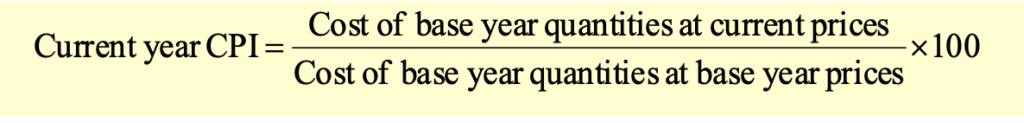# Nominal Vs. Real GDP

At times, we would like to compare the GDP data in different time periods. Since the average level of prices of goods and services change over time, the GDP data may not be directly comparable. We will need to make some adjustments to differentiate a nominal change in GDP from a real change in GDP.

A nominal change in GDP is a change in GDP that occurs only due to the change in price levels. Nominal GDP is based on current prices. This is the GDP that we described in the nominal approach.A real change in GDP reflects the actual increase in the physical quantity of output. This is done by adjusting the GDP for inflation. It measures the current year output using the prices from a base year.### Price Indexes

The nominal GDP can be converted into real GDP using a price index. A price index is a weighted average of the prices of a set of the goods and services produced in the economy over a period of time. Two commonly used price indexes are GDP deflator and Consumer Price Index (CPI)

#### Implicit GDP Price Deflator

The implicit GDP price deflator is calculated as follows:#### Consumer Price Index

It is a fixed-weight price index, which takes the quantities in some base year as being the typical goods bought by the average consumer during that base year, and then uses those quantities (same basket) as weights to calculate the index in each year.# Checkout our eBooks and Templates

eBooks and templates related to finance, R programming, Python, and Excel.
Visit Store
Get our Data Science for Finance Bundle for just $29$51. That's 43% OFF.
Get it for $51$29